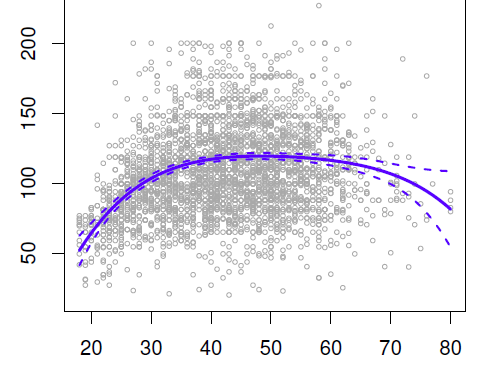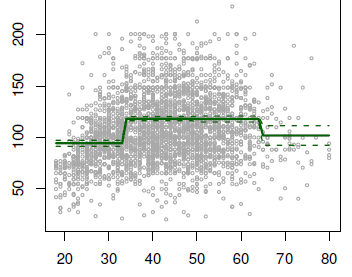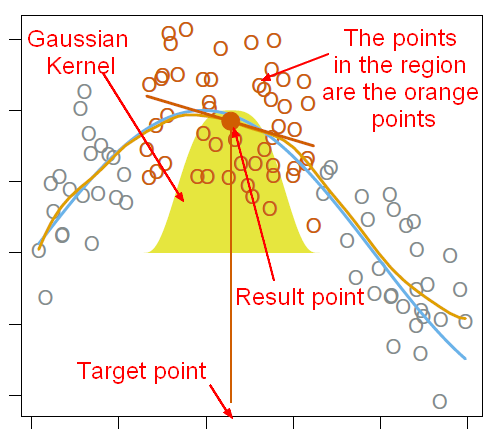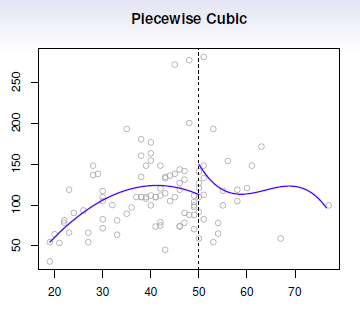# Data Mining - Global vs Local

Global refers to calculation that are made over the whole data set whereas local refers to calculations that are made local to a point or a partition.

## High dimension vs Local

In high dimension, it's really difficult to stay local.

Example: the percentage of volume that contains 10% of the data in an hypercube is:

• Two dimension: $1^2 - 0.9^2 = 1 - 0.81 = 0.19 = 19\%$
• Ten dimensions: $1^{10} - 0.9^{10} = 1 - 0.35 = 0.65 = 65\%$

To resolved this problem, (structured|parametrized) model have been introduced. The simplest one is the linear model.

## Documentation / Reference

Recommended PagesData Mining - (Global) Polynomial Regression (Degree)

polynomials regression Although polynomials are easy to think of, splines are much better behaved and more local. With polynomial regression, you create new variables that are just transformations...Data Mining - Dimensionality (number of variable, parameter) (P)

Not to confound with d: the model size. You may have 1000 attributes (p=1000) in your sample but after feature selection for instance, you model may use only a handful (d=5) In physics and mathematics,...Data Mining - High Dimension (Curse of Dimensionality)

High dimension In high dimension, it's really difficult to stay local. edge cases See this interactive app in R Shiny on the Curse of Dimensionality. Circle...Data Mining - Step Function (piecewise constants)

Step functions, are another way of fitting non-linearities. (especially popular in epidemiology and biostatistics) Continuous variable are cut into discrete sub-ranges and fit a constant model in...Statistics - Knots (Cut points)

Knots are cutpoints that defines different regions (or partitions) for a variable. In each regions, a fitting must occurs. The definition of different regions is a way to stay local in the fitting process....Statistics - LOcal (Weighted) regrESSion (LOESS|LOWESS)

Popular family of methods called local regression that helps fitting non-linear functions just focusing locally on the data. LOESS and LOWESS (locally weighted scatterplot smoothing) are two strongly...Statistics - Piecewise polynomials

Piecewise polynomials generalize the idea of piecewise constants. The idea try to get rid of the global polynomial because it's global and not local. Instead of having asingle polynomial over the whole...